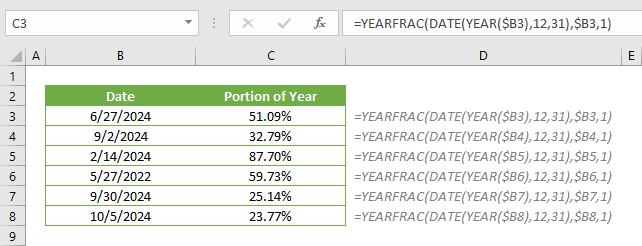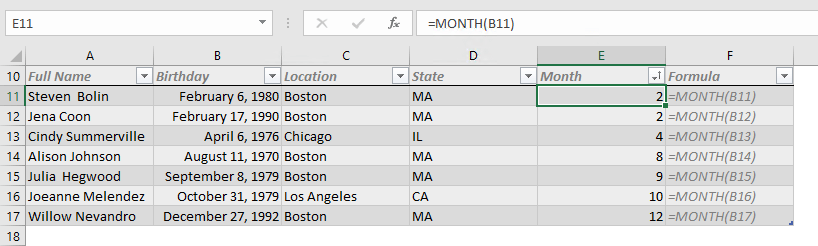Select Page## How to count the number of cells greater than the average in Excel

In this article, we are going to show you how to count the number of cells greater than the average in Excel. Download Workbook Formula =COUNTIFS(<numbers range>,”>“&AVERAGE(<numbers range>)) <numbers range>: The reference from the range of...## How to count the number of cells less than the average in Excel

In this article, we are going to show you how to count the number of cells that is less than the average of that data set in Excel. Download Workbook Formula =COUNTIFS(<numbers range>,”<“&AVERAGE(<numbers range>)) <numbers range>: The...## How to calculate the percent of completed year

A percentage value is a great way to express a proportion’s size in whole. You may want to calculate and see what percent of the year is completed. In this article, we are going to show you how to calculate the percent of completed year in Excel. Download Workbook...## How to sort dates by month in Excel

Sorting is a crucial part of organizing data. Being everyone’s go-to spreadsheet tool, Excel thankfully offers various tools for sorting data like the sort feature and functions like SORT or SORTBY. However, neither of these approaches can really help you with...## How to create an Excel summary table using UNIQUE and SUMIFS

SUMIFS like other …IF or …IFS function are great tools to aggregate data based on a set of conditions. The downside of this approach is that the criteria must be supplied manually, especially when you need to create a summary table. In this guide, we’re going to show...